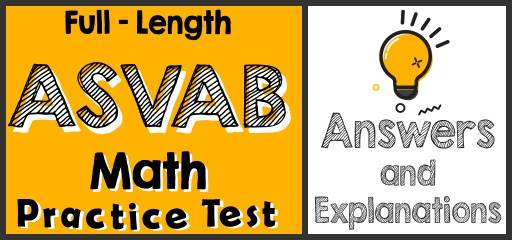# Full-Length ASVAB Math Practice Test-Answers and ExplanationsDid you take the ASVAB Math Practice Test? If so, then it’s time to review your results to see where you went wrong and what areas you need to improve.

## ASVAB Mathematical Reasoning Practice Test Answers and Explanations

1- Choice C is correct
Each worker can walk 2 dogs: $$8 ÷ 4 = 2$$, 3 workers can walk 6 dogs. $$3 × 2 = 6$$

2- Choice A is correct
Change 6 hours to minutes, then: $$6 × 60 = 360$$ minutes, $$\frac{360 }{90}= 4$$

3- Choice A is correct
The base rate is $20. The fee for the first 40 visits is: $$40×0.25=10$$ The fee for the visits 41 to 60 is:$$20×0.15=3$$, Total charge: $$20 + 10 + 3 = 33$$ 4- Choice C is correct 12 dozen of magazines are 144 magazines: $$12 × 12 = 144, 144 – 36 = 108$$ 5- Choice C is correct 1 ton $$= 2,000$$ pounds, 3ton $$= 6,000$$ pounds, $$\frac{27,000}{6,000} = 4.5$$ William needs to make at least 5 trips to deliver all of the food. 6- Choice A is correct average$$=\frac{sum}{total}=\frac{28+23+36}{3}=\frac{87}{3}=29$$ 7- Choice B is correct Find the value of each choice: $$A. 2×2×5×7= 140$$ $$B. 2×2×2×5×7= 280$$ $$C. 2×7= 14$$ $$D. 2×2×2×2×5×7= 560$$ 8- Choice D is correct Karen $$=$$Michelle $$+ 8$$, Michelle $$=$$David $$– 5$$, Karen $$+$$ Michelle $$+$$ David $$=88$$ Michelle $$=$$David $$– 5 ⇒$$ David $$=$$Michelle$$+ 5$$, Karen $$+$$ Michelle $$+$$ David $$=88$$ Now, replace the ages of Karen and David by Michelle. Then: Michelle $$+ 8 +$$ Michelle $$+$$ Michelle $$+ 5 =88$$, 3Michelle $$+ 13 =88$$ ⇒ 3Michelle $$=88 – 13$$ 3Michelle $$=75$$, Michelle $$= 25$$ ## The Absolute Best Book to Ace the ASVAB Math Test 9- Choice A is correct Area of a rectangle $$=$$ width $$×$$ length $$= 25 × 50 = 1,250$$ 10- Choice C is correct The amount they have $$= 11.25 + 13.50 + 16.45 = 41.20$$ 11- Choice D is correct distance$$=$$speed $$×$$time $$⇒$$time$$=\frac{distanc}{speed}=\frac{400}{40}=10$$ (Round trip means that the distance is 400 miles) The round trip takes 10 hours. Change hours to minutes, then: $$10×60=600$$ 12- Choice B is correct $$200 – 50 – 55 = 95$$ ## Best ASVAB Math Prep Resource for 2022 13- Choice C is correct average$$=\frac{sum}{total}$$ Sum $$= 5 + 8 + 13 + 18 + 22 + 25 + 28 + 25 = 144$$ Total number of numbers $$= 8$$ average$$=\frac{144}{8} = 18$$ 14- Choice B is correct Since Julie gives 6 pieces of candy to each of her friends, then, the number of pieces of candies must be divisible by $$6. 190 ÷ 6 = 31.67, 240 ÷ 6 = 40, 260 ÷ 6 = 43.33, 275 ÷ 6 = 45.83$$, Only choice b gives a whole number. 15- Choice A is correct probability$$=\frac{desird outcomes}{possible outcomes}=\frac{3}{4+3+7+10}=\frac{3}{24}=\frac{1}{8}$$ 16- Choice B is correct $$30 ÷ 2 = 15$$ hours for one course, $$15 × 22 = 330⇒ 330$$ ## The Most Comprehensive Workbook for the ASVAB Math test ## More from Effortless Math for ASVAB Test … ### Need great ASVAB Math worksheets to help you learn basic math concepts in a structured manner? Use our ASVAB Math Worksheets for the ASVAB math preparation. We prepared a complete list of the top 10 free websites for the ASVAB exam to help you pass this exam. Also, you can use our ASVAB Math Formulas to ace the ASVAB math subtests. ## The Best Books to Ace the ASVAB Math Test ## Have any questions about the ASVAB Test? Write your questions about the ASVAB or any other topics below and we’ll reply! ## Related to This Article ### More math articles ### What people say about "Full-Length ASVAB Math Practice Test-Answers and Explanations - Effortless Math: We Help Students Learn to LOVE Mathematics"? No one replied yet. X 52% OFF Limited time only! Save Over 52% SAVE$40

It was $76.99 now it is$36.99